• // 编写C++程序完成以下功能： //（1） 假定矩阵大小为4×5（整型数组表示）； //（2） 定义矩阵初始化函数，可以从cin中输入矩阵元素； //（3） 定义矩阵输出函数，...//（8） 计算并输出：A3 = A1A2，A3 = A1A2。
• 稀疏矩阵加减乘C++实现 可以编译！！！！！！！！！！
• 主要介绍了Java实现矩阵加减乘除及转制等运算功能,结合实例形式总结分析了java常见的矩阵运算实现技巧,需要的朋友可以参考下
•jar
• 本代码实现CUDA中矩阵加减以及转置的运算 （总共2个CPP 1个H 1个CU 1个CUH文件）
• 文章目录matlab 矩阵加减乘除运算1 加、减运算2 乘法**3．向量点积****4．向量叉乘****5．混合积****6．矩阵的卷积和多项式乘法**7．反褶积（解卷）和多项式除法运算8．张量积**9 除法运算** matlab 矩阵加减乘除...


文章目录
matlab 矩阵加减乘除运算1 .加、减运算2. 乘法3．向量点积4．向量叉乘5．混合积6．矩阵的卷积和多项式乘法7．反褶积（解卷）和多项式除法运算8．张量积9. 除法运算

matlab 矩阵加减乘除运算
1 .加、减运算
运算符：“＋”和“－”分别为加、减运算符。 运算规则：对应元素相加、减，即按线性代数中矩阵的“十”，“一”运算进行。
2. 乘法
运算符：* 运算规则：按线性代数中矩阵乘法运算进行，即放在前面的矩阵的各行元素，分别与放 在后面的矩阵的各列元素对应相乘并相加。
3．向量点积
函数 dot 格式 C = dot(A,B) %若 A、B 为向量，则返回向量 A 与 B 的点积，A 与 B 长度 相同；若为矩阵，则 A 与 B 有相同的维数。 C = dot(A,B,dim) %在 dim 维数中给出 A 与 B 的点积
例 >>X=[-1 0 2]；
>>Y=[-2 -1 1];
>>Z=dot(X, Y)
则显示：Z =
4
还可用另一种算法：
sum(X.*Y)
ans=
4

4．向量叉乘
在数学上，两向量的叉乘是一个过两相交向量的交点且垂直于两向量所在平面的向量。 在 Matlab 中，用函数 cross 实现。 函数 cross 格式 C = cross(A,B) %若 A、B 为向量，则返回 A 与 B 的叉乘，即 C=A×B，A、B 必须是 3 个元素的向量；若 A、B 为矩阵，则返回一个 3×n 矩阵，其中的列是 A 与 B 对应列的叉积，A、B 都是 3×n 矩 阵。 C = cross(A,B,dim) %在 dim 维数中给出向量 A 与 B 的叉积。A 和 B 必须具有 相同的维数，size(A,dim)和 size(B,dim)必须是 3。 例 1-24 计算垂直于向量(1, 2, 3)和(4, 5, 6)的向量。
 >>a=[1 2 3];
>>b=[4 5 6];
>>c=cross(a,b)
结果显示：
c=
-3 6 -3
可得垂直于向量(1, 2, 3)和(4, 5, 6)的向量为±(-3, 6, -3)

5．混合积
混合积由以上两函数实现： 例 计算向量 a=(1, 2, 3)、b=(4, 5, 6)和 c=(-3, 6, -3) 的混合积a ⋅(b ×c) 解：
>>a=[1 2 3]; b=[4 5 6]; c=[-3 6 -3];
>>x=dot(a, cross(b, c))
结果显示：x =
54
注意：先叉乘后点乘，顺序不可颠倒。

6．矩阵的卷积和多项式乘法
函数 conv 格式 w = conv(u,v) %u、v 为向量，其长度可不相同。 说明 长度为 m 的向量序列 u 和长度为 n 的向量序列 v 的卷积(Convolution)定义为： ∑= = + − kj 1 w (k) u(j) v(k 1 j) 式中：w 向量序列的长度为(m+n-1)，当 m=n 时， w(1) = u(1)*v(1) w(2) = u(1)*v(2)+u(2)*v(1) w(3) = u(1)*v(3)+u(2)*v(2)+u(3)*v(1) … w(n) = u(1)*v(n)+u(2)*v(n-1)+ … +u(n)v(1) … w(2n-1) = u(n)*v(n)
7．反褶积（解卷）和多项式除法运算
函数 deconv 格式 [q,r] = deconv(v,u) %多项式 v 除以多项式 u，返回商多项式 q 和余多项式 r。 注意：v、u、q、r 都是按降幂排列的多项式系数向量。
8．张量积
函数 kron 格式 C=kron (A,B) %A 为 m×n 矩阵，B 为 p×q 矩阵，则 C 为 mp×nq 矩阵。
9. 除法运算
Matlab 提供了两种除法运算：左除（\）和右除（/）。一般情况下，x=a\b 是方程 ax =b 的解，而 x=b/a 是方程 xa=b 的解。
例：a=[1 2 3; 4 2 6; 7 4 9]
b=[4; 1; 2];
x=a\b
则显示：x=
-1.5000
2.0000
0.5000
如果 a 为非奇异矩阵，则 a\b 和 b/a 可通过 a 的逆矩阵与 b 阵得到：
a\b = inv(a)*b
b/a = b*inv(a)

数组除法： A./B 表示 A 中元素与 B 中元素对应相除
资料整理来源：[MATLAB6.0数学手册].蒲俊.吉家锋.伊良忠
展开全文matlab
• 实现两个矩阵加减乘计算，， import javax.swing.*; public class Matrix extends JApplet{ public void init(){ String rowString1,//第一个矩阵的行数，用作输入用 rankString1,//第一个矩阵的列数，用作...
• 稀疏矩阵是指那些多数元素为零的矩阵。利用“稀疏”特点进行存储和...实现一个能进行稀疏矩阵基本加减运算的运算器。 【基本要求】 稀疏矩阵的表示采用三元组，而运算结果的矩阵则以通常的阵列形式和三元组形式列出。
• 稀疏矩阵加法、减法、乘法运算，抱歉没有除法运算，vc6.0运行
• 矩阵求逆时借鉴了helloworld07的求逆算法，不过把他的C算法稍加改变成C++语言的形式。在这里谢谢他！
• 两个不同维度的矩阵相加规则： 1.若两个矩阵对应维度不同，则应使其中一个矩阵的维度为1，则会自动广播。 如：a = (3,4,5,6) ;b=(1,4,5,6);c=a+b=(3,4,5,6) a = (3,4,1,6) ;b=(1,4,5,6);c=a+b=(3,4,5,6) 2.若两...
两个不同维度的矩阵相加规则：
1.若两个矩阵对应维度不同，则应使其中一个矩阵的维度为1，则会自动广播。
如：a = (3,4,5,6) ;b=(1,4,5,6);c=a+b=(3,4,5,6)
a = (3,4,1,6) ;b=(1,4,5,6);c=a+b=(3,4,5,6)
2.若两个矩阵维度不同，则从后往前数：对应维度相同的和多余出来的维度为最终维度。
如：a = (3,4,5,6) ;b=(4,5,6);c=a+b=(3,4,5,6)
a = (3,4,5,6) ;b=(1,5,6);c=a+b=(3,4,5,6)
a = (3,5,1,7) ;b=(1,4,7);c=a+b=(3,5,4,7)
展开全文• 稀疏矩阵的十字链表表示方法：矩阵加减乘法运算、矩阵转置运算、矩阵项的插入、矩阵行列链表的排序
• ## 矩阵加减 乘法的C语言实现

万次阅读 多人点赞 2018-10-11 17:08:30
矩阵减法：就是对应位置相加即可 对于满足以下条件的矩阵A，B： 我们有： 加法， 减法，     矩阵的乘法： 对于满足以下条件的矩阵A，B，C： 我们有： 其中：   要注意的是...
矩阵的加减法：就是对应位置相加减即可
对于满足以下条件的矩阵A，B：

我们有：
加法，

减法，

矩阵的乘法：
对于满足以下条件的矩阵A，B，C：

我们有：

其中：

要注意的是矩阵群没有乘法交换律，也就是说：

下面的代码是输入两个矩阵，输出他们的乘
#include <stdio.h>

int main(void)
{
int mTemp, rTemp, nTemp;
scanf("%d %d %d", &mTemp, &rTemp, &nTemp);
const int m = mTemp, r = rTemp, n = nTemp;	//注意数组的声明要用常数
double A[m][r], B[r][n], C[m][n];

//输入A，B两个矩阵
for (int i = 0; i <= m - 1; i++) {
for (int j = 0; j <= r - 1; j++) {
scanf("%lf", &A[i][j]);
}
}
for (int i = 0; i <= r - 1; i++) {
for (int j = 0; j <= n - 1; j++) {
scanf("%lf", &B[i][j]);
}
}

//将矩阵（二维数组）C 的所有元素全部初始化为零
for (int i = 0; i <= m - 1; i++) {
for (int j = 0; j <= n - 1; j++) {
C[i][j] = 0;
}
}

//矩阵乘法
for (int i = 0; i <= m - 1; i++) {
for (int j = 0; j <= n - 1; j++) {
for (int k = 0; k <= r - 1; k++) {
C[i][j] += A[i][k] * B[k][j];
}
}
}

//输出结果
for (int i = 0; i <= m - 1; i++) {
for (int j = 0; j <= n - 1; j++) {
printf("%10.5f ", C[i][j]);
}
printf("\n");
}

return 0;
}


当我们要进行计算的时候，首先输入m, r, n，再输入矩阵A， 再输入矩阵B
比如，我想要计算一下这两组矩阵

我就要这样输入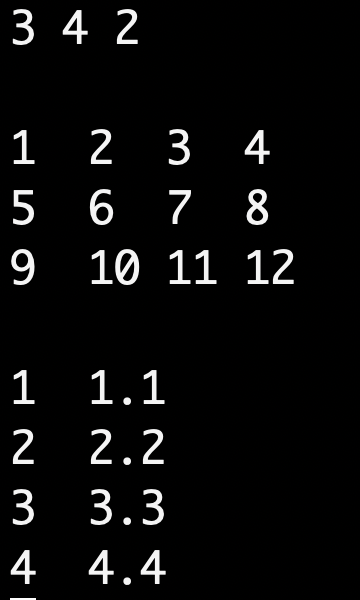输出结果：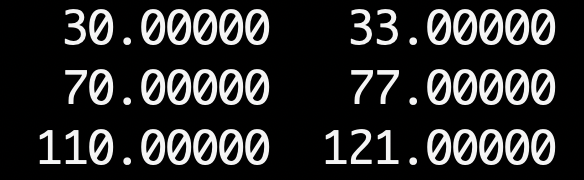发现精度都没用上，居然是整数，那就再算一组，来个复杂点的

输入：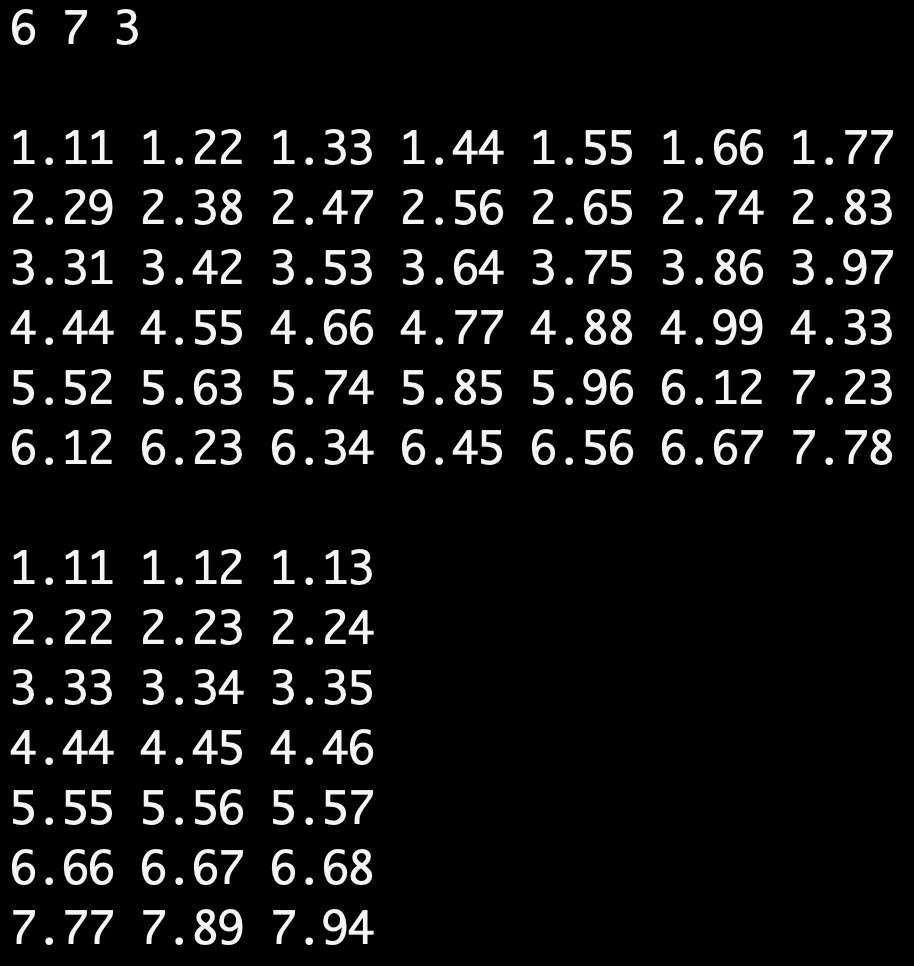输出：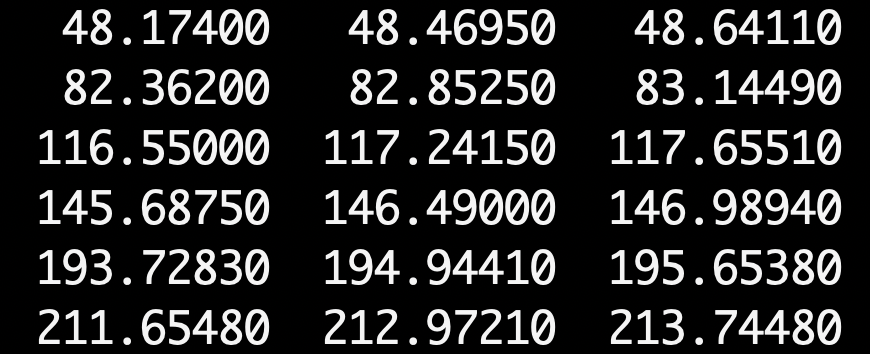展开全文C语言
• VC6.0编译。详细注释。稀疏矩阵的连续存储空间表示：矩阵加减乘法运算、矩阵转置运算、矩阵项的插入、矩阵行列链表的排序
• 实现两个稀疏矩阵求和相减，相乘操作。 [基本要求] （1）矩阵可键盘输入。（2）输出求和，相减，相乘结果； （3）利用三元组数据结构。
• 矩阵加法就是相同位置的数字一下。 矩阵减法也类似。 矩阵乘以一个常数，就是所有位置都乘以这个数。 但是，等到矩阵乘以矩阵的时候，一切就不一样了。 这个结果是怎么算出来的？ 教科书告诉你，计算...
矩阵加法就是相同位置的数字加一下。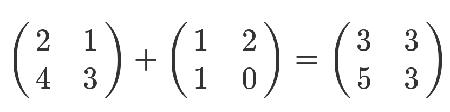矩阵减法也类似。
矩阵乘以一个常数，就是所有位置都乘以这个数。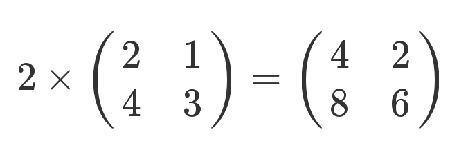但是，等到矩阵乘以矩阵的时候，一切就不一样了。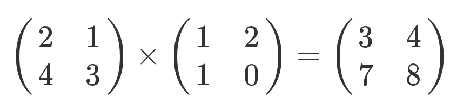这个结果是怎么算出来的？
教科书告诉你，计算规则是，第一个矩阵第一行的每个数字（2和1），各自乘以第二个矩阵第一列对应位置的数字（1和1），然后将乘积相加（ 2 x 1 + 1 x 1），得到结果矩阵左上角的那个值3。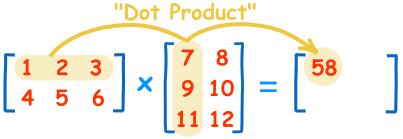也就是说，结果矩阵第m行与第n列交叉位置的那个值，等于第一个矩阵第m行与第二个矩阵第n列，对应位置的每个值的乘积之和。
应用：蓝书P418 例3
展开全文• 1、矩阵加减乘除求逆运算的概念： 　（1）矩阵概念  有m n个数排列成一个m行n 列，并括以方括弧（或圆括弧）的数表称为m行n 列矩阵。 　（2）矩阵加法：   （3）矩阵乘法： 　（4）矩阵的求逆运算 　...
• 项目中经常遇到不同维度矩阵加减操作，规则如下： （1）进行加减操作的两个矩阵最后一个维度要相同或其中一个矩阵的最后一个维度元素数为1 a=np.array([[1,2],[3,4]]) print(a.shape) b=np.array([[[7,8],[8,9]], ...numpy python 机器学习 深度学习
• 几个简单的矩阵运算的模板类。有加减乘和求逆运算。
• 参数平差，矩阵，参数平差，矩阵，参数平差，矩阵
• 稀疏矩阵是指那些多数元素为零的矩阵。利用“稀疏”特点进行存储（只存储非零元）和计算可以大大节省存储空间，提高计算效率。实现一个能进行稀疏矩阵基本运算的运算器。 二、需求分析： 以“带行逻辑链接信息”的...
• struct matrix { int m; int w,h; matrix(int x,int y) { h=x,w=y; memset(m,0,sizeof(m)); } matrix() { memset(m,0,sizeof(m)); } void in
• 网上down到的 但是自己的编程基础较差不太会用
• 矩阵运算系统可以完成矩阵、乘法，但是只限于方阵，其编译环境为VC6.0，经过反复验证，完全正确。本程序没有在TURBOC上验证其正确性。
•C代码
• 用C#实现了矩阵的相加、相减、相乘、求逆、转置等功能！希望能帮到大家！
• 基于MFC的代码，实现矩阵加减乘除，求逆等运算,在CEditView中实现。...